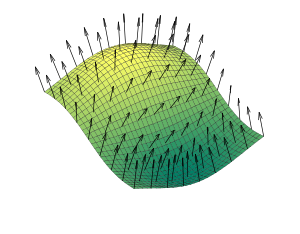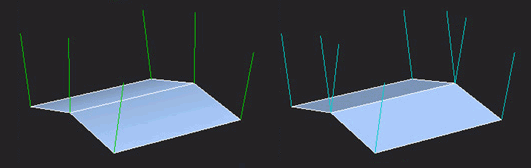Hi,

I have a terrain and I am trying to write a shader in order to have exactly this result:

This is easily possible when we have unique triangles (vertices not being shared with other triangles), but I have large terrains and for performance purposes I try to limit that. The problem is the default interpolation behavior which results in that:

The solution seems to be in dealing with HLSL but it would be even better if you have any other.

I tried several things with effects:

• interpolation modifiers (nointerpolation for normals), which seems ignored
• specifying the ShadeMode to flat in the pass, which seems unsupported

I really searched for hours, but did not find satisfying results, I would be glad if you have even an idea.

Thank you.

this is not trivial with the limited amount of vertices (as you said not 4 per quad).

Interpolation works, but it will yield triangles (still interesting look) try it

In your vertex output struct you can define

struct DrawTerrain_VSOut
{
float4 Position : SV_POSITION0;
nointerpolation float4 Color : COLOR0;

};

for color or for normals.
I used this for my game a while back - it looks like thsiHope this helps

This is the result I now get:

With that:

``````struct VertexShaderInput
{
float4 Position : SV_POSITION0;
float4 Normal : NORMAL0;
float4 Color : COLOR0;
};
{
float4 Position : SV_POSITION0;
float4 worldPos : TEXCOORD0;
nointerpolation float4 Color : COLOR0;
};

{

float4 worldPosition = mul(input.Position, xWorld);
float4 viewPosition = mul(worldPosition, xView);

output.Position = mul(viewPosition, xProjection);
output.worldPos = worldPosition;
output.Color = input.Color;

return output;
}

{
float3 n = input.worldPos;
float3 dndx = ddx(n);
float3 dndy = ddy(n);
float3 norm = -normalize(cross(dndx, dndy));

float LightingFactor = dot(norm, -xLightDirection);

float4 output = input.Color;
output.rgb *= saturate(LightingFactor) + xAmbient;

return output;
}
``````

The nointerpolation has still no effect as you see, am I doing something wrong?

I tried that also, no change.
I am on OSX, but the render is the same on Windows.

Your nointerpolation image looks correct, it’s working as intended.

in your pixel shader you can do this for better non-interpolated normals.

float3 normal = normalize(cross(ddy(input.WorldPosition.xyz), ddx(input.WorldPosition.xyz)));

Interpolation is still quite visible, I really don’t understand why that nointerpolation has no effect.
Anyways, a temporary solution might be to not have brutal color changes.
This is my result with some noise:

Has anybody a better solution?

Your picture looks like it’s working as intended (again)

if you set nointerpolation to your normals (or use the formula given above) you can change the lighting from

ndl = saturate(dot(lightVector, normal))
light = ndl;

to

light = ndl*ndl;

to achieve a more dramatic change of gradient

Alright, thanks a lot.

Ya the actual solution is that you pretty much need indices to shared vertices and each vertice needs it own calculated normal based on some surrounding basis variable.

Though ill say it’s trivial in concept to explain this.
It tends to be non trivial to implement.
Even the simplest implementations take a bit of effort.

Basically.

You need to generate each vertice normal based on all the other surrounding shared vertice normal’s or triangle planes, or some set mapping normal say per quad that pertains to each quad itself.

With nurbs you have a control net of points that creates the terrain and can create the normals at once. however with just the normals there are a couple of fairly simple ways to do it basically by looping and averaging but you still have to sort of build a sort of control net, but this is a lot easier then it sounds.

Now there are a few ways to do this ill list One of them.

Edit listed below in couple posts down.

This is a post processing step for terrain before you shade it.

Probably not the best picture for this. These are pretty huge patches but each vertice normal is calculated separately. So even on large triangles with jagged edges, the lighting still smooths out across adjoining triangles for each normal.
For example if you look at the lower left base of the first large bump on the right the shadowed area is dead on a vertice and extends outwards to all the triangles from it, this is also proportionally c0 continuous curvature. So the quads sharing vertices in a index buffer and normal’s are basically necessary.
Unless you want to update 3 vertices normals per triangle redundantly or intentionally want sharp irreglar lighting on a specific area.

I had actually planned to create a little community nurbs terrain editor that i was working on in xna but thats sort of on the backburner.

that is the condition though

It’s also possible without that condition just not so easily.

Actually its the same either way just more complicated looping and storage with each triangle draw separately.

like what im saying is to generate the normals you need the white squares shown in my picture to be “something” but you need them specifically. Those in principal whatever you base them off of are your base values for a basis function to generate smooth contiguous shared or not shared vertice normals for proper lighting.This is what you have going on your case is shown on the right.
You have to create it by combining and smoothing the normal’s out before you pass them.
You have 2 quads with two triangles each, you have 2 sets of 4 normal’s and 6 vertices.
you must create a smooth adjoining normal yourself, as shown on the leftYou solve this directly per vertice by calculating the surrounding triangles around each vertice directly calculating those cross products and averaging then normalizing the result and placing it as the current vertices surface normal you must be cautious of the calculation in regards to winding order.

or .

In the case of quad terrain to generate the lighting normal’s properly for a simple array, not a nurb surface like in my picture, its still the same idea. Those may based off each grid or quads total normals that surround any single vertice.

You need a single normal for each whole quad found from all his current surrounding triangle normal’s this can be simply found per quad in his specific case. In order to reform those current vertice normals and get rid of the discontinuous artifacts.

Then he needs to regenerate each vertice normal by what is essentially averaging the quad normals (he just found created), back to each vertice normal, this requires a bit of effort but that is basically the simple gist of it.

To say it another way…

Each quad needs to use all 4 of its vertices or both its triangles to create a per quad normal basis.
Each vertice needs to have its four surrounding quad base normals to be regenerated into to a smooth normal.

So basically each vertice normal requires the influence of the 8 surrounding vertice normals to be regenerated this can be based directly off the triangles as well were either way, this is normally a pre-shader operation done on the vertices themselves before passing to the gpu for terrain just once when you create the terrain itself.

However edge cases that are out of bounds must not be sumed or they will error out if this is wraped around such as a sphere.
Then if the index to be found if it is more then L will be by index = index - L if less then zero index = index + L;

if you really want to make it “hardcoded” into the normal you can just calculate the normal of the quad, assign it to the first vertex in the quad, make both triangles have this vertex indexed as first and use nointerpolation and it works without multiple vertices per quad.

That said, the ddx ddy thing is easy and reliable and you don’t have to calculate normals at all and just pass position to the vertex buffer

The problem is when you assign normal’s to a vertice array that is indexed just creating a normal based on a triangle means the next triangle is getting a improper normal or the last one.
I don’t know how flat shading works but if it smooths then its gotta be doing something similar on the card itself. Though i cant imagine it being cheaper then pre-calculating the vertice normals just once beforehand.

I had the code to automate this for quads but i have no idea were i buried it but.

This looks about right it doesn’t worry about out of bounds positions as it just searches the indexs.
As well it uses the pre-created winding order for each triangle it finds with the vertice your checking.
It’s not fast but it only has to occur once you could save and load the resulting output.
If your terrain changes or morphs while the game runs, you’'ll have to recalculate the vertices involved.

``````        // un-tested
public VertexPositionNormalTexture[] CreateSmoothNormals(VertexPositionNormalTexture[] vertices, int[] triangles)
{
for (int i = 0; i < vertices.Length; i++)
{
var n = vertices[i].Position;
Vector3 average = Vector3.Zero;
float total = 0;
for (int j = 0; j < triangles.Length; j++)
{
var c = triangles[j];
if (c == i)
{
var s = (int)(c / 3) * 3;
var v0 = triangles[s];
var v1 = triangles[s + 1];
var p0 = vertices[v0].Position;
var p1 = vertices[v0].Position;
average += Vector3.Cross(p0, p1);
total += 1;
}
}
if (total > 0)
{
var newnormal = average / total;
newnormal.Normalize();
vertices[i].Position = newnormal;
}
}
return vertices;
}
``````

Try that after you set up your terrain.

It should give you smooth shading as its calculating all actual surrounding triangle planes around a vertice.

I think @kosmonautgames’ solution is better and you’re probably messing something upbut let’s throw another idea in here!

You can use instanced rendering to solve the problem. Basically you can have multiple vertex streams that go to their next vertex at a different frequency (after a different number of actual vertices drawn). That means you can have a second vertex stream that updates every six triangles and has a vertex per quad. To do this you need a second vertexbuffer that holds the quad normals and set its frequency value to 6 (6 vertixes per quad). Compute the normal for each quad, put it in the vertex buffer, use SetVertexBuffers on your graphicsdevice and that’s it! No need to change stuff in the shader (from what you originally had, so explicitly passing the normal).

Side note: this is currently only implemented for DX, but a working pull request is awaiting merge.

you can use the same normal for 2 triangles if they both start with the same vertex